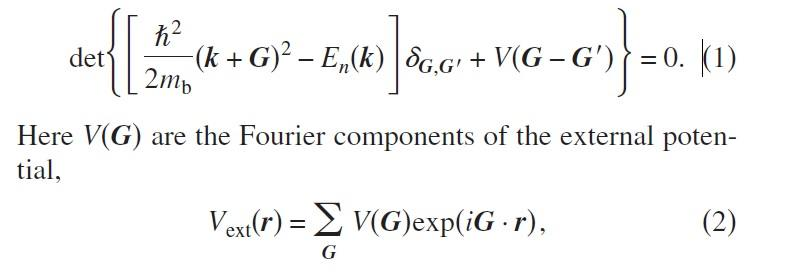# Bloch theory on hexagonal lattice

• A
frankzappa
Hello, i am new in condensed matter physics and studying the article 'Engineering artificial graphene in a two-dimensional electron gas' (Gibertini et al., 2009). I read a lot about bloch theorem lately and i got the main idea but still i don't understand the component of the equation. In the article they used 2D muffin-tin potential with ##r=52.5nm## and the center to center distance between the disk is ##a=150nm## and band mass is ##m_b=0.067m## and ##V_0=1.0 meV##.I'm trying to solve this equation numerically in Matlab. I created 2x100 matrices of ##\mathbf{G}## by the formula:
$$\mathbf{G}= l\mathbf{g_1}+p\mathbf{g_2}$$
with ##\mathbf{g_1}=\frac{2\pi(1,\sqrt 3)} {3a}## and ##\mathbf{g_2}=\frac{2\pi(1,-\sqrt 3)} {3a}##. But i don't know to how to formulize any other elements. For example i have tried to find the ##\mathbf{k}## with $$\mathbf{k}=\sum_{j=1}^2 \frac {m_j} {N_j} \mathbf{G_j}$$ However i didnt succeed.

How can i study to this topic and solve the equation?

What you are trying to do is not easy.

Any good book on solid-state physics will have many chapters covering what you need to know (Beginner: Band Theory and Electronic Theory of Solids by Singleton. Intermediate: Kittel. Advanced: Ashcroft & Mermin). In fact, the article you mention cited Ashcroft & Mermin.

When you say you are trying to solve the equation, you are actually trying to calculate the electronic band structure: ##E_n(\vec k)##. We call ##\vec k## the crystal momentum (technically it is a quantum number). In the reduced-zone scheme, it runs from 0 to the zone boundary. The subscript ##n## is another quantum number that labels which band you are in; it is analogous to three of the four quantum numbers you see in atoms.

You should be aware that they've already plotted three electronic band structures in Figure 1 of the paper.

frankzappa
Thank you for your answer. I'am trying to reproduce Figure 1 in the paper in a manner of better understanding. I have read related topics from Ashcroft & Mermin and Michael P. Marder's books but still i am not able to do numerical calculations.
After i created 2x100 matrices for ##\mathbf G## i tried to writed down ##\mathbf k## as;
$$\mathbf k= \frac 1 {100} [(\frac {2 \pi} {3a} + \frac {2 \pi} {3a}, \frac {2\pi \sqrt{3}} {3a} - \frac {2\pi \sqrt{3}} {3a}]$$
$$\mathbf k = \frac 1 {100} [\frac {4 \pi} {3a}, 0]$$
This one doesn't make sense to me because of 0.
Also i didn't understand the muffin-tin potential. I am still studying the books and they make sense when read their texts but i stuck everytime i try to solve something numerically.

Last edited:
Thank you for your answer. I'am trying to reproduce Figure 1 in the paper in a manner of better understanding it. I have read related topics from Ashcroft & Mermin and Michael P. Marder's books but still i am not able to do numerical calculations. After i created 2x100 matrices for ##\mathbf G## i tried to writed down ##\mathbf k## as;
$$\mathbf k= \frac 1 {100} [(\frac {2 \pi} {3a} + \frac {2 \pi} {3a}, \frac {2\pi \sqrt{3}} {3a} - \frac {2\pi \sqrt{3}} {3a}]$$
$$\mathbf k = \frac 1 {100} [\frac {4 \pi} {3a}, 0]$$
This one doesn't make sense to me.
I was a little sloppy in my first reply regarding the crystal momentum ##\vec k##. Unfortunately, I think this is something you don't understand.

When we calculate electronic band structures they are displayed in reciprocal space. This name comes from the fact that the zone boundaries are calculated by taking the reciprocal of lattice vectors. The ##\vec k## vectors fill all of reciprocal space just like position vectors ##\vec r## fill all of real space.

I'm not sure on this point, but it appears you are trying to calculate a reciprocal lattice vector ##\vec G## when you say you are calculating ##\vec k##.

Since you haven't mentioned a wave function, I don't think you realized how hard this is going to be. In Ashcroft & Mermin, you should read past the section on muffin-tin potentials. The next two sections on the augmented plane-wave method (APW) and the Green's function method of KKR show you what you need to be doing.

But all this hard work has been done before. There are many programs (most are not free) that calculate electronic band structures for you.

All that being said, what are you trying to learn about this system? Perhaps, I can guide you to an easier way.

•frankzappa
frankzappa
Thanks for your help. My first goal was write down the components of equation. For this i define ##\mathbf G=l\mathbf g_1 + p\mathbf g_2 ## in a loop for ##l## and ##p## from 1 to 10 and created a 2x100 matrices. As i understand ##\mathbf G## is like translation vector ##\mathbf T## in reciprocal space. So with this i have define a crystal with 10 unit cell in direction ##\mathbf g_1## and 10 unit cell in the direction of ##\mathbf g_2## i guess. And ##N_1=10## and ##N_2=10##. Then
$$\mathbf k = \sum_{j=1}^{2} \frac {m_j} {N_j} \mathbf g_j = \frac {1} {10} (m_1 \mathbf g_1+ m_2 \mathbf g_2)$$

About ##m_j##, I have question marks in my head but this is how i tried to calculate the ##\mathbf k##

I didn't read the read the chapter 11 yet, I'm going to try to understand it. Thanks for the lead.

Last edited: